# 100 percent hypothesis

The 100% Hypothesis for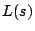asserts that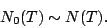Here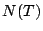is the zero counting function for, and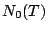counts only the zeros on the critical line. In other words, 100 percent of the nontrivial zeros (in the sense of density) are on the critical line. An equivalent assertion is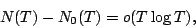which makes it clear that the 100% Hypothesis still allows quite a few zeros off the critical line.

The term 100% Hypothesis'' is not standard.

In contrast to most of the other conjectures in this section, the 100% Hypothesis is not motivated by applications to the prime numbers. Indeed, at present there are no known consequences of this hypothesis.

Back to the main index for The Riemann Hypothesis.